### 3.3.1 Establishing a bijection between tuples and integers

Consider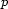domains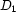through. Each domainhas cardinality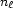:The cardinality of the cartesian product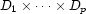is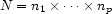. We are going to establish a bijection betweenand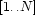.

The idea is to partition the intervalintoequal subintervals: one for each value in. Then to partition each subinterval into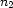subsubintervals: one for each value in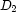. Etc recursively.

It is easy to capture this idea in a formula. Consider a tuple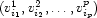. According to the recursive algorithm outlined above, it is assigned to the following integer: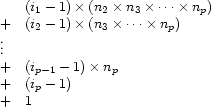Thus, given an indexin the range, we can recover the corresponding tuple by calling {DecodeInt I-1 Divs Doms}, where Divs is the list: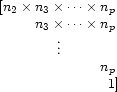and Doms is the list of domains, each one consisting of a list of values. The function DecodeInt is implemented as follows:

fun {DecodeInt I Divs Doms}   case Divs#Doms   of nil#nil then nil   [] (Div|Divs)#(Dom|Doms) then       Q = I div Div      R = I mod Div   in       {Nth Dom Q+1}|{DecodeInt R Divs Doms}   end end

1. actually we can recover this distinction easily as explained in Section 3.6.

Denys Duchier
Version 1.2.0 (20010221)